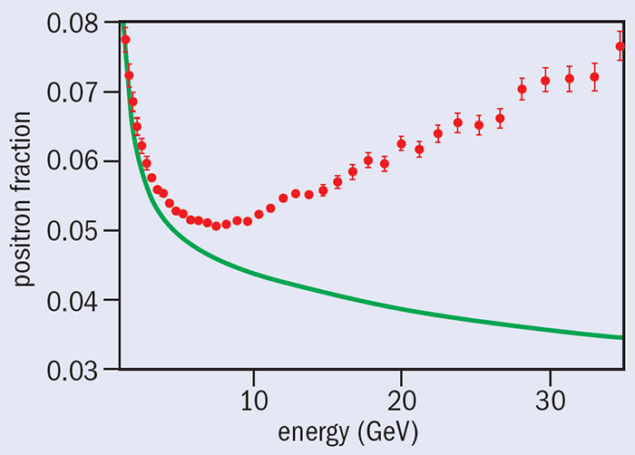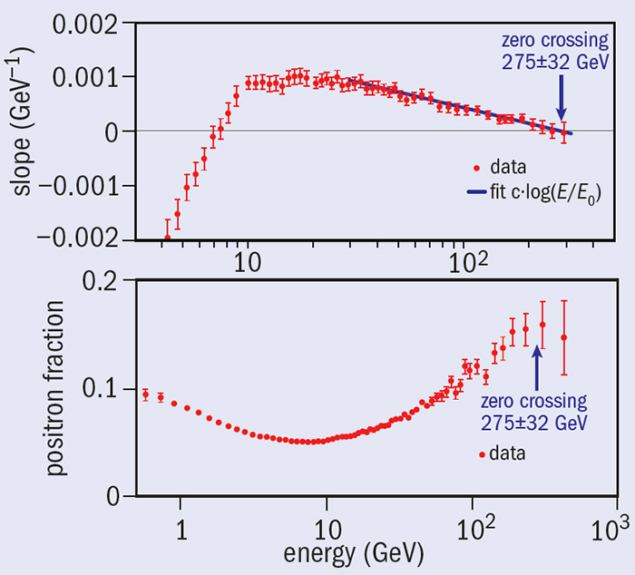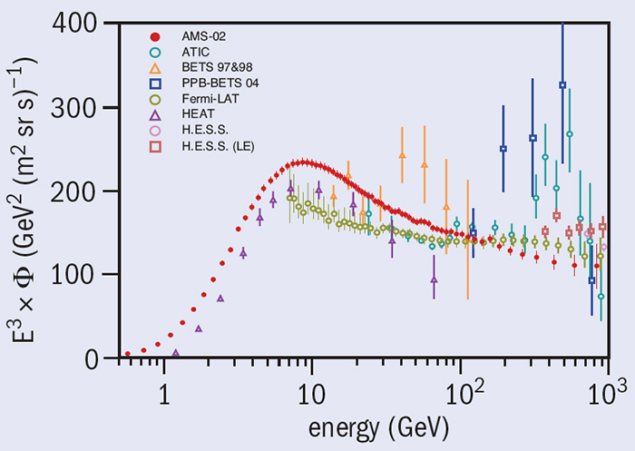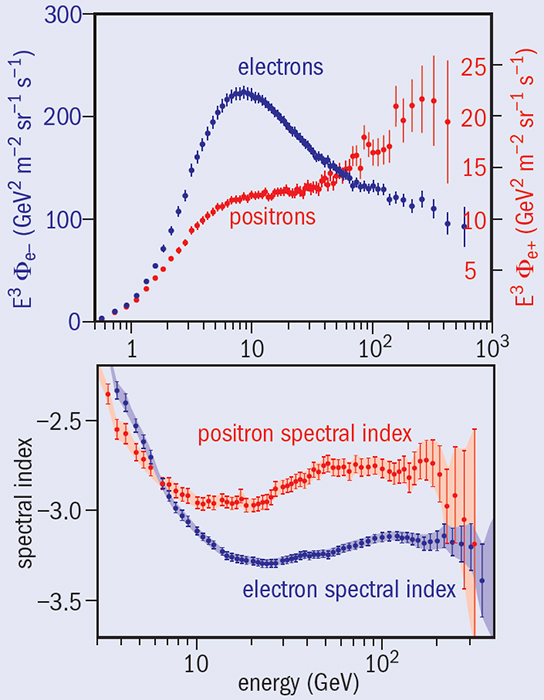# AMS finds evidence of new source of positrons in cosmic rays

27 October 2014Fig. 1. The positron fraction measured by AMS (red circles) compared with the expectation from ordinary cosmic-ray collisions. The rapid rise at 8 GeV indicates a new source of positrons.

The Alpha Magnetic Spectrometer (AMS) on the International Space Station (ISS) has new results on energetic cosmic-ray electrons and positrons, based on analysis of the first 41 billion events. These results provide a deeper understanding of the nature of high-energy cosmic rays and could shed more light on the existence of dark matter.

Of the 41 × 109 primary cosmic-ray events analysed so far, 10.9 × 106 have been identified as electrons and positrons. Using these, the AMS collaboration has measured the positron fraction – the ratio of the number of positrons to the combined number of positrons and electrons – in the energy range 0.5–500 GeV (Accardo et al. 2014). When compared with the expectation based on the production of positrons in standard cosmic-ray collisions, the results show that the fraction starts to increase rapidly at 8 GeV (figure 1). This indicates the existence of a new source of positrons.Fig. 2. Top: the slope of positron fraction measured by AMS (red circles) and a straight line fit at the highest energies (blue line). Bottom: the measured positron fraction as a function of energy.

AMS has also accurately determined the exact rate at which the positron fraction increases with energy, and for the first time observed the fraction reach a maximum (figure 2). The data show that the rate of change of the positron fraction crosses zero at 275±32 GeV – indicating the energy at which the fraction reaches its maximum (Aguilar et al. 2014). The results also show that the excess of the positron fraction is isotropic within 3%, suggesting strongly that the energetic positrons might not be coming from a preferred direction in space. Moreover, the fraction shows no observable sharp structures.Fig. 3. AMS measurement of the electron flux (red dots), compared with results from other experiments.

AMS has also precisely determined the flux of electrons (figure 3) as well as for positrons (figure 4). These measurements reveal that the fluxes differ significantly in both their magnitude and energy dependence. The positron flux first increases (0.5–10 GeV) and then levels out (10–30 GeV), before increasing again (30–200 GeV). Above 200 GeV, it has a tendency to decrease. This is totally different from the scaled electron flux. The results show that neither flux can be described with a constant spectral index (figure 4, bottom). In particular, between 20 and 200 GeV, the rate of change of the positron flux is surprisingly higher than the rate for electrons. This is important proof that the excess seen in the positron fraction is from a relative excess of high-energy positrons, and not the loss of high-energy electrons.Fig. 4. Top: AMS measurements of the electron flux (left scale) and the positron flux (right scale). Bottom: the spectral indices of the fluxes as functions of energy.

Different models for dark matter predict different behaviours for the positron-fraction excess. The new results from AMS put much tighter constraints on the validity of these models. The results are consistent with a dark-matter particle (neutralino) of mass of the order of 1 TeV. To determine if the observed new phenomenon is indeed from dark matter or from astrophysical sources such as pulsars, AMS is now making measurements to determine the rate at which the positron fraction decreases beyond the turning point, as well as to determine the antiproton fraction.

• Fifteen countries from Europe, Asia and America participated in the construction of AMS: Finland, France, Germany, the Netherlands, Italy, Portugal, Spain, Switzerland, Turkey, China, Korea, Taiwan, Russia, Mexico and the US. AMS was launched by NASA to the ISS on 16 May 2011. Data are transmitted to the AMS Payload Operations Control Center, located at CERN.